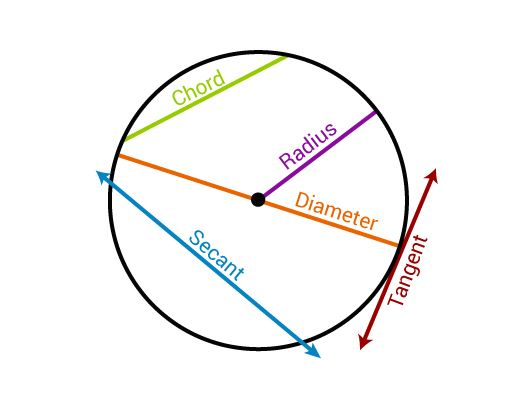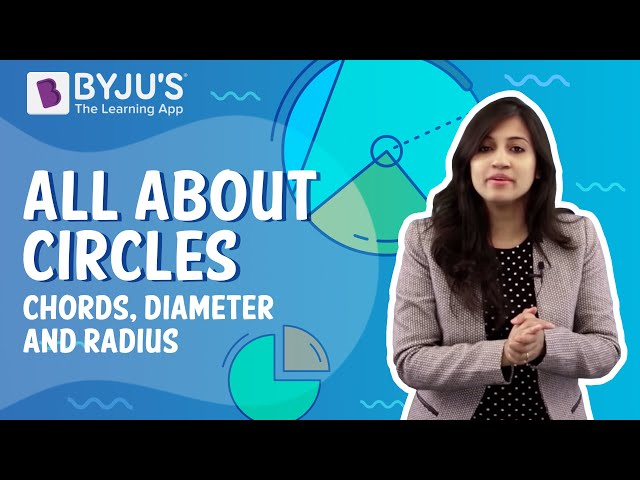# Secant of a Circle

To learn about the Secant of a circle, let’s recall what a circle is. A circle is a closed loop. In a circle, every point on the circle is at an equal distance from the centre O. In a secant, the line intersects the circle at two points. But a tangent intersects the circle at only one point around its outer line. This is the basic difference between a secant and a tangent. But the common fact between them is both the lines are drawn from outside of the circle. Let us learn more here.

## Secant of the Circle Definition

A straight line that intersects a circle in two points is called a secant line. A chord is the line segment that joins two distinct points of the circle. A chord is in a unique secant line and every secant line defines a unique chord. In geometry, a secant is a line that cuts any curve in at least two different points. Secant means ‘to cut’ extracted from a Latin word ‘secare’. While in a circle, a secant will touch the circle in exactly two points and a chord is the line segment defined by these two points, that is the interval on a secant whose endpoints are these two points.

### Secant of a Circle Formula

If a secant and a tangent of a circle are drawn from a point outside the circle, then;

Lengths of the secant × its external segment = (length of the tangent segment)2

### Diameter of Circle – Secant

A secant is an extension of a chord in a circle which is a straight line segment of which the endpoints lie on the circle. If the same chord passes through the centre of the circle, then it is a diameter. So an extended Diameter is a secant.

## Intersecting Secants

When two secants of a circle intersect each other at a point outside the circle, there becomes an intersecting relationship between those two line segments.

If PQ and RS are the intersecting secants of the given circle then ( P + Q). Q = (R + S) .SIn the above figure, you can see:

• Blue line segment is the secant
• Red shows the tangent
• Green is the chord of circle

### How do you find the secant of a circle?

Secant intersects the circle exactly at two points. The measure of an angle formed by a tangent and a secant or two secants or two tangents which intersect outside the circle is one half the positive difference of the measures of the intercepted arcs.

### Difference between a secant and a chord

A part of a line between the two endpoints is called a line segment.

 Secant Chord It intersects the circle at two points It touches the circle at two points It is drawn from outside the circle It lies within the circle

## Video Lessons on Circles

### Introduction to Circles### Parts of a Circle### Area of a Circle